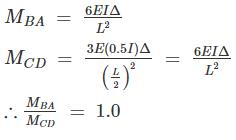Courses

# Theory Of Structures 1 MCQ

## 20 Questions MCQ Test Mock test series of SSC JE Civil Engineering | Theory Of Structures 1 MCQ

Description
This mock test of Theory Of Structures 1 MCQ for Civil Engineering (CE) helps you for every Civil Engineering (CE) entrance exam. This contains 20 Multiple Choice Questions for Civil Engineering (CE) Theory Of Structures 1 MCQ (mcq) to study with solutions a complete question bank. The solved questions answers in this Theory Of Structures 1 MCQ quiz give you a good mix of easy questions and tough questions. Civil Engineering (CE) students definitely take this Theory Of Structures 1 MCQ exercise for a better result in the exam. You can find other Theory Of Structures 1 MCQ extra questions, long questions & short questions for Civil Engineering (CE) on EduRev as well by searching above.
QUESTION: 1

Solution:
QUESTION: 2

Solution:
QUESTION: 3

### If ∑H and ∑V are the algebraic sums of the forces resolved horizontally and vertically respectively, and ∑M is the algebraic sum of the moments of forces about any point, for the equilibrium of the body acted upon.

Solution:

For equilibrium of any body.

(i) ∑FH = 0

(ii) ∑FV = 0

(iii) ∑MZ = 0

QUESTION: 4

The force in CD of the truss shown in Fig, is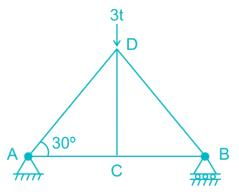Solution:
QUESTION: 5

A two hinge arch of span 40 m carries a point load of 62.8 KN at its crown. The Horizontal thrust in the arch is (in KN)

Solution:
QUESTION: 6

What is the degree of static indeterminacy of the structure shown in figure below?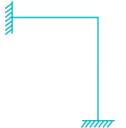Solution:
QUESTION: 7

If a system has more equations of equilibrium than no. of forces, then the system is:-

Solution:

Let Ds = static Indeterminacy

Ds = R - r

R → no. of unknowns

r → No. of equilibrium equations available

If r > R

Ds < 0, system is partially constraintIf

Ds > 0, then system is over stiff.

QUESTION: 8

Which of the following material is not used in making trusses?

Solution:

Concrete is a brittle material and is unable to take tension. In Truss both tension and compression force is available, that’s why concrete is not used in making truss. While metal bars and channels are made of material which have both tensile as well as compressive strength.

QUESTION: 9

What is the major difference between truss and beam?

Solution:

Beam are the horizontal members which can transmit the load perpendicular to their longitudinal direction and not in axial direction while truss can transmit the load in axial direction only.

QUESTION: 10

The Muller-Breslau principle in structure analysis is used for

Solution:

Muller Breslau Principle: The Muller-Breslau principle states that the ILD for any stress function in a structure is represented by its deflected shape obtained by removing the restrained offered by that stress function and introducing a directly related generalised unit displacement in the direction of that stress function.

QUESTION: 11

The influence line diagram for bending moment at 6 m is shown. The value of the maximum bending moment is: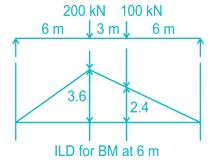Solution:

The value of maximum bending moment is = 200 x 3.6 + 100 x 2.4 = 960 kN-m

QUESTION: 12

The degree of static indeterminacy of the pin - jointed plane truss as shown in figure is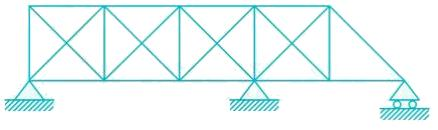Solution:

Ds = m – 2j + re

m = 23

j = 11

∴ Ds = 23 – 22 + 5 = 6

QUESTION: 13

Due to rising temperature in a three hinged arch, induced stress is:

Solution:

Three-hinged arch is a determinate structure and rise in temperature do not produce any stress.

QUESTION: 14

Which of the following equals the number of unknowns to be determined, in stiffness method of structural analysis?

Solution:

Force method is useful when Ds < Dk

Displacement method is useful when Dk < D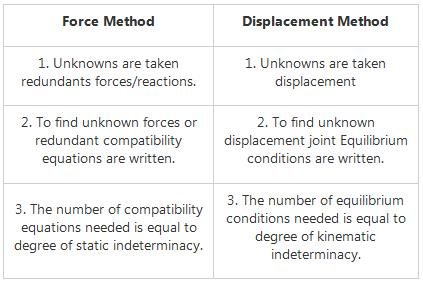QUESTION: 15

The unit load method used in structural analysis is

Solution:

The unit load method is extensively used in the calculation of deflection of beams, frames and trusses. Theoretically this method can be used to calculate deflections in statically determinate and indeterminate structures. However it is extensively used in evaluation of deflections of statically determinate structures only as the method requires a priori knowledge of internal stress resultants. The unit load method used in structural analysis is derived from Castigliano’s theorem.

QUESTION: 16

The degree of kinematic indeterminacy of the given beam considering axial rigidity is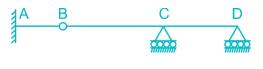Solution:

Dk = 3j – re + rr

= 3 x 4 – 5 + 1 = 8

If members are considered axially rigid

Dk = 8 – 3 = 5

QUESTION: 17

Consider the following statements:

The principle of superposition is not applicable when

1. The material does not obey Hooke’s law

2. The effect of temperature changes are taken into consideration

3. The structure is being analysed for the effect of support settlement

Which of these statement (s) is/are correct?

Solution:

According to the principle of superposition, for a linearly elastic structure, the load effects caused by two or more loadings are the sum of the load effects caused by each loading separately. Note that the principle is limited to:

• Linear material behaviour only;

• Structures undergoing small deformations only (linear geometry).

It is not applicable when:

1. The material does not obey Hooke’s law.

2. The effect of temperature changes are taken into consideration.

3. The structure is being analysed for the effect of support settlement.

QUESTION: 18

Force in the member BC of the truss shown in the given figure is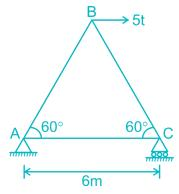Solution:
QUESTION: 19

Clapeyron’s theorem is associated with the analysis of

Solution:
• Beams that have more than one span are defined as continuous beams. Continuous beams are very common in the bridge and building structures.
• When a beam is continuous over many supports and the moment of inertia of different spans is different, the force method of analysis becomes quite cumbersome.
• However, the force method of analysis could be further simplified for this particular case (continuous beam) by choosing the unknown bending moments at the supports as unknowns.
• One compatibility equation is written at each intermediate support of a continuous beam in terms of the loads on the adjacent span and bending moment at left, center (the support where the compatibility equation is written) and rigid supports.
• Two consecutive spans of the continuous beam are considered at one time. Since the compatibility equation is written in terms of three moments, it is known as the equation of three moments.
• In this manner, each span is treated individually as a simply supported beam with external loads and two end support moments.
• For each intermediate support, one compatibility equation is written in terms of three moments. Thus, we get as many equations as there are unknowns. Each equation will have only three unknowns.
• Clapeyron first proposed this method in 1857 and it is widely known as the Clapeyron theorem.
QUESTION: 20

The given figure shows a portal frame with one end fixed and other hinged. The ratio of the fixed end moments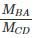due to side sway will be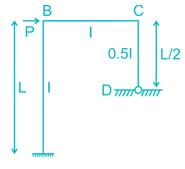Solution:

Due to sway, the deflection of point B will be equal to that of point C.